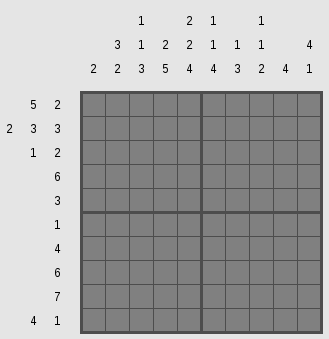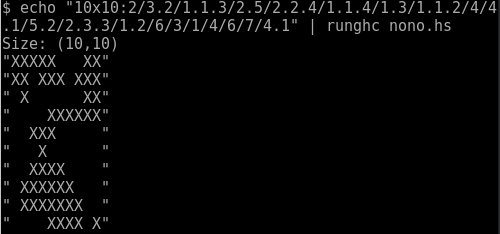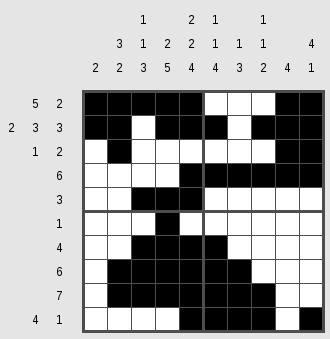Published: Sun 31 January 2016

# Solving Tatham's Puzzles - Pattern

I've started writing solvers for games of Simon Tatham's Portable Puzzle Collection. Those Problems can usually be reduced to some NP-complete problem. This is quite a fun exercise.

We will be starting with the puzzle "Pattern", also known as Nonograms that we reduce to SAT.Unsolved Nonogram

First to explain the puzzle: The cells of the puzzle can be filled with black or white squares. The numbers on the sides of the rows and colums denote the number of consecutive black squares. Every run of black squares has to be seperated from another run by white squares.

First we want to define a type for our problem.

```type PSize = (Int, Int)
type Run = [[Int]]
type Runs = (Run, Run)
data Problem = Problem PSize Runs deriving Show
```

The puzzle on the side is defined in the Tatham format by the string 10x10:2/3.2/1.1.3/2.5/2.2.4/1.1.4/1.3/1.1.2/4/4.1/5.2/2.3.3/1.2/6/3/1/4/6/7/4.1. It has a size information and then the list of runs. Another format we want to support is the Haskell Runs format which would be ([,[3,2],[1,1,3],[2,5],[2,2,4],[1,1,4],[1,3],[1,1,2],,[4,1]],[[5,2],[2,3,3],[1,2],,,,,,,[4,1]]). This format seperates the runs for rows and columns and ommits the size information.

For the second format we can use Haskell's own read function. For Tatham's format we have to define a parser. I've added type information for most functions which is often needed for code involving read.

```import Text.ParserCombinators.ReadP
number' = many1 (choice (map char ['0'..'9']))

r_size = do l <- number'
char 'x'
r <- number'

r_run = do { li <- sepBy number' (char '.'); return \$ map read li }

r_runs = sepBy r_run (char '/')

r_problem = do size <- r_size; char ':'
runs <- r_runs
skipSpaces; eof
return \$ Problem size (take (fst size) runs, drop (fst size) runs)
```

What do we now do with such a problem? First we want to check it for consistency. If we got size information it should fit the runs and the numbers in the runs (and the spaces between them) shouldn't add up to more than the size.

```import qualified Prelude as P (all, (&&), not)
consistent :: Problem -> Bool
consistent (Problem size (xin, yin)) = consistent' size xin P.&& consistent' (swap size) yin
consistent' (w, h) xs = (w == length xs) P.&& P.all (\x -> (sum x) + (length x - 1) <= h) xs
```Calling our solver

But now on to solving the problem. We will reduce this problem to SAT using the Ersatz library. The encoding of a problem, I guess, is obvious. We say that every cell within the Nonogram is a Variable Bit and a bit being True has the semantics of that field being colored black while False would mean white.

We want to build constraints of single rows or columns and then solve the conjunction of all those row/column-constraints for satisfiability. Therefore we define a function of the type [Int] -> [Bit] -> Bit that takes a list of Integers (the numbers of one column or row) and the list of remaining Bits that do not yet are constrained.

First we begin with the end of the recursion. If no number is left or the remaining number is zero (for empty columns or rows), we want all remaining variables to be False. (we take for to be defined as flip map)

```build_runs :: [Int] -> [Bit] -> Bit
build_runs nums vars = case nums of
[] -> all not vars
 -> all not vars
x:xs -> let max_d = length vars - (length xs + sum xs) - x
run   = [not] ++ replicate x id ++ [not]
v     = [false] ++ vars ++ [false]
in any id \$ for [0..max_d] \$ \ d ->
(all not \$ take d vars)
&& (all id \$ zipWith id run \$ take (x + 2) \$ drop d v)
&& (build_runs xs \$ drop (x + d + 1) vars)
```Solved Nonogram

The recursion is something to think about a bit. We're given a number of consecutive black squares x and all the variables in the column/row vars. The x Trues can be put anywhere from 0 to max_d where max_d is all the rest of the variables take the minimum necessary space of the remaining number of numbers xs, while all variables before that number of black squares and one after need to be white. For every possible position the rest of the variables need to fulfill the same condition with the rest of the numbers.

Now we have to combine all the constraints from a given problem.

```nono :: (MonadState s m, HasSAT s) => Problem -> m (Map (Int,Int) Bit)
nono (Problem (sizex, sizey) (xin, yin)) = do
let indices = [(x, y) | x <- [1..sizex], y <- [1..sizey] ]
vars' <- replicateM (length indices) exists
vars <- return \$ Map.fromList \$ zip indices vars'
let getx x = for [1..sizey] \$ \y -> (vars ! (x,y))
gety y = for [1..sizex] \$ \x -> (vars ! (x,y))
xs = zipWith build_runs xin (map getx [1..])
ys = zipWith build_runs yin (map gety [1..])
assert (all id xs && all id ys)
return vars
```

Internally we let Ersatz identify variables by their position in the grid Map (Int,Int) Bit. Now we can solve our problem easily using minisat with Ersatz's native minisat binding. Given a solution, we just format it correctly and give it to the user.

```main = do
input <- getContents
let parsed = parse_tat input
problem = case parsed of
Just p -> p
Nothing -> let (xin, yin) = read input :: ([[Int]],[[Int]])
in Problem (length xin, length yin) (xin, yin)

putStrLn \$ "Size: " ++ (show \$ problem_size problem)
unless (consistent problem) \$ do {putStrLn "Problem inconsitent"; exitFailure}

(Satisfied, Just solution) <- solveWith minisat (nono problem)
let sizey = snd \$ problem_size problem
lines = for [1..sizey] \$ \i -> filter (\ ((x, y), b) -> y == i) \$ toList solution
conv  = map \$ \((x,y), b)-> if b then 'X' else ' '
join = foldr (:) ""
forM_ (map (join . conv) lines) print
```

And we're done.

Find the code here: github.com/maweki/tatham-slv

This post is part of the series "Tatham's Puzzles":

Comments and Discussion is provided by Disqus. They are tracking site and user interaction. Please refer to their privacy policy for information about data usage and retention. If you still want to look at comments or comment yourself, enable disqus by clicking here.

Previous: Gnome Foundation membership renewed , Next: My computer science course of Jan 2016